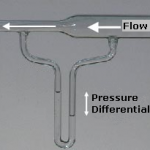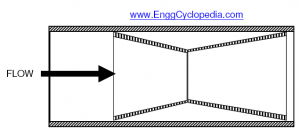# Pressure based flow measurement devices

Flow measuring devices can be primarily classified based on the type of flow measurement - either mass flow measurement or volumetric flow measurement. The flow measurement devices can also be further classified based on their principle of operation. Here a list and brief explanation of commonly used flow measurement devices is presented.

## Pressure drop based flow measurement devicesOrifice plate is a plate with an orifice which restricts the flow, thereby causing a pressure drop which is related to the volumetric flow based on Bernoulli's equation. The pressure drop across the orifice plate is measured and the corresponding volumetric flow can be calculated using Bernoulli's equation. Orifice plate causes high energy losses and high pressure loss to the flow being measured. Typically flow coefficient which represents the ease of flow to pass through, is equal to 0.62 for orifice plates.

Venturi meter is also based on Bernoulli's principle similar to orifice plate. But instead of sudden constriction caused by an orifice, venturi meter uses a relatively gradual constriction much like a reducer to cause the pressure drop by increasing fluid velocity. The volumetric flow is proportional to the square root of this pressure drop and venturi meter can be calibrated accordingly. Venturi meter is responsible for lower pressure and energy losses compared to orifice plates. Typical flow coefficient for venturi meter ranges between 0.93 to 0.97 indicating easier passage of flow.Dall tubes are in between venturi meters and orifice plates in terms of flow coefficients. They are shorter than venturi meters but have less pressure drop than orifice plates. Due to low pressure losses they are preferred over orifice plates to be used in lines with large flowrates.Pitot Tubes is a pressure measuring instrument used to estimate fluid velocity by measuring the pressure in the developed fluid flow. Bernoulli's equation is then used to calculate the fluid velocity based on measured value of pressure. Pitot tube measures the pressure at one point in the fluid flow across the cross section. This pressure value can be used to calculate only one dimensional velocity at that particular point using the Bernoulli's equation.

Multihole pressure probe is a more sophisticated version of a pitot tube. This multihole pressure probe can perform pressure measurement at multiple points in the cross sectional fluid flow profile. These multiple values of pressure at different points in the cross section can then be used to estimate a 2 dimensional or 3 dimensional velocity vector based on number of holes used in the probe. The theory behind estimating velocity is same as the one used for pitot tube and is based on Bernoulli's equation.